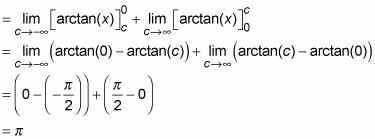##### Finite Math For DummiesOne of the ways in which definite integrals can be improper is when one or both of the limits of integration are infinite. You solve this type of improper integral by turning it into a limit problem where c approaches infinity or negative infinity. Here are two examples: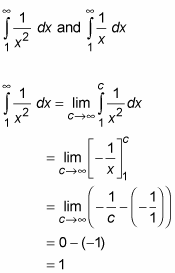Because this improper integral has a finite answer, you say that it converges.

Convergence and Divergence: An improper integral converges if the limit exists, that is, if the limit equals a finite number. Otherwise, an improper integral is said to diverge.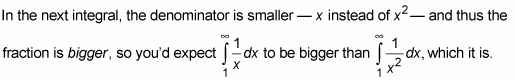But it’s not just bigger, it’s way, way bigger.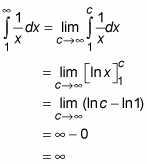This improper integral diverges.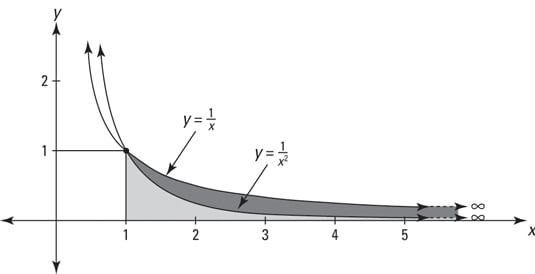The figure above shows these two functions.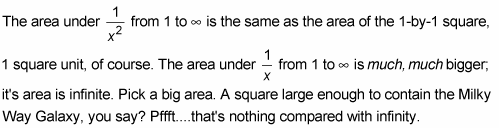Their shapes are quite similar, but their areas couldn’t be more different.

Let’s move on to something slightly more complicated. When both of the limits of integration are infinite, you split the integral in two and turn each part into a limit. Splitting up the integral at x = 0 is convenient because zero’s an easy number to deal with, but you can split it up anywhere you like. Zero might also seem like a good choice because it looks like it’s in the middle between negative infinity and infinity. But that’s an illusion because there is no middle between negative infinity and infinity, or you could say that any point on the x-axis is the middle.

Here’s an example: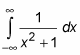1. Split the integral in two.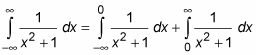2. Turn each part into a limit.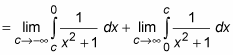3. Evaluate each part and add up the results.